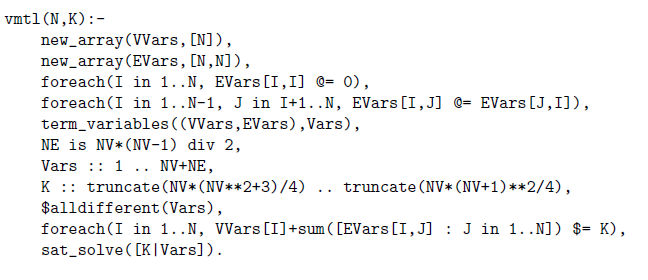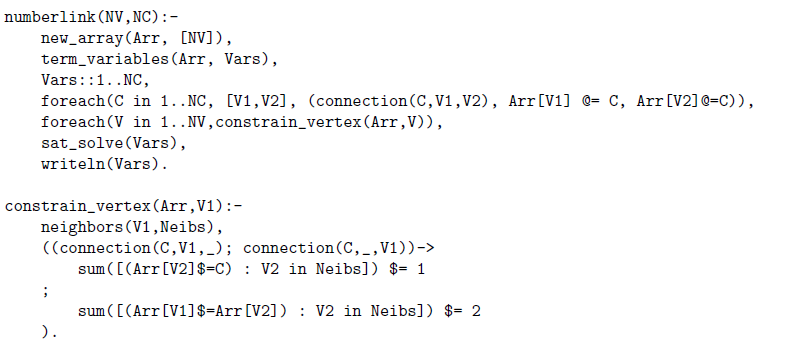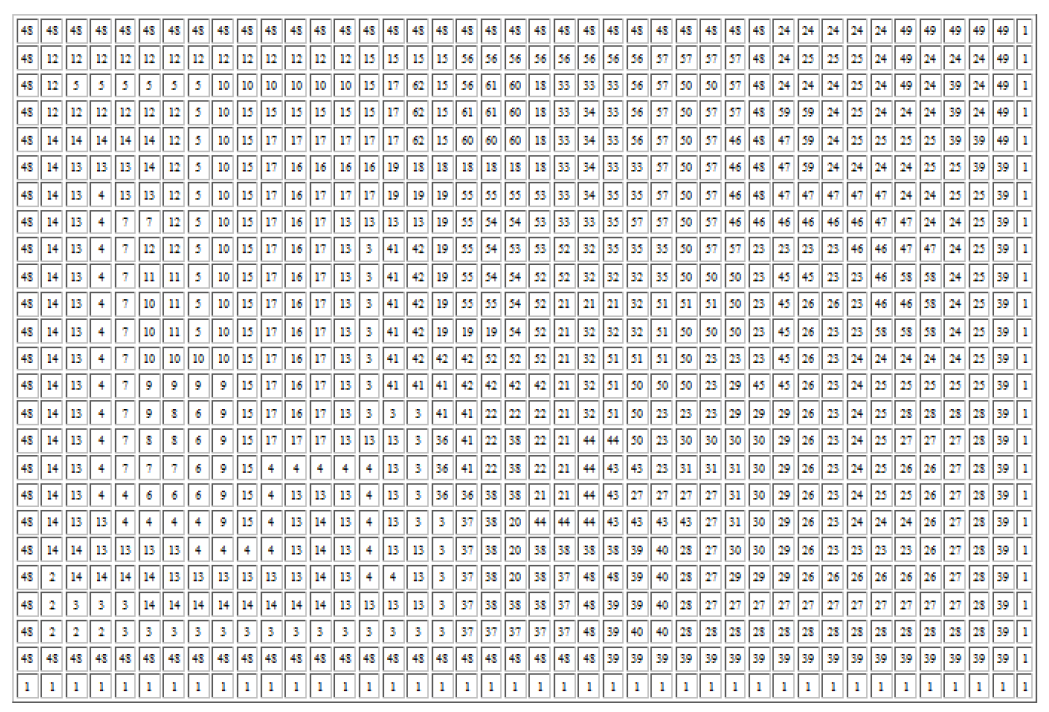# The SAT Compiler in B-Prolog

By

### Neng-Fa Zhou

CUNY Brooklyn College & Graduate Center

### 1 Introduction

Several systematic search methods, including Dynamic Programming (DP) , Constraint Programming (CP) , Linear Programming & Mixed Integer Programming(LP/MIP) , and Boolean satisfiability (SAT) , are available for solving combinatorial search problems. DP relies on memoization of solutions to subproblems to avoid redundant computations. CP uses constraint propagation to prune search spaces and heuristics to guide search. IP relies on LP relaxation and branch-and-cut to find optimal integer solutions. SAT performs unit propagation and clause learning to prune search spaces, and employs heuristics and learned clauses to do non-chronological backtracking. No method is superior all the time. DP is useful when many subproblems have the same or similar structures and can share solutions. CP is well suited to problems for which global constraints, symmetry breaking, and problem-specific propagation and labeling can be exploited. IP tends to be well suited to problems that can be naturally expressed with disequality constraints. SAT is suited to not only problems that are intrinsically Boolean but also non-Boolean problems for which heuristics cannot be easily programmed. Recent advancement of SAT has been the key to the success of Answer Set Programming .

With tabling for dynamic programming, CLP(FD), and an interface to LP/MIP solvers, B-Prolog provides a set of useful tools for solving combinatorial search problems. Recently, the toolbox has been enriched with a SAT compiler, which compiles constraint programs into SAT. The B-Prolog interface to SAT comprises primitives for creating decision variables, specifying constraints, and a built-in, called sat_solve, for invoking a solver. The same interface is also used for LP/MIP and CP solvers.

The implementation of the interface makes use of attributed variables in B-Prolog to accumulate constraints. When a constraint is posted, it is added to the list of accumulated constraints. Only when a solver-invoking call is executed, are the constraints interpreted. If the solver is CP invoked by cp_solve, then the accumulated constraints are added into B-Prolog’s constraint store and a labeling predicate is called to start the search. If the solver is SAT, then all the variables are Booleanized and all the constraints are sent to the SAT solver after being compiled into CNF. If the solver is LP/MIP invoked by lp_solve or ip_solve, then all the constraints are converted to disequalities before being sent the LP/MIP solver. An answer found by the solver is returned to B-Prolog as bindings of the decision variables.

The B-Prolog SAT compiler employs the so called log-encoding for compiling domain variables and constraints . The same encoding has also been used by the Minizinc SAT compiler . Log-encoding has less propagation power than direct and support encodings for certain constraints , but is much more compact than other encodings, including the order encoding which is used by the Sugar  and the BEE  compilers.

### 2 Examples

This section gives two example programs in B-Prolog to illustrate the usage of the SAT compiler. In the examples, the built-in sat_solve is used to invoke the SAT solver. This built-in can be replaced by ip_solve to call the IP solver, or by cp_solve to call the CP solver. These examples use some of the non-standard features of B-Prolog, such as arrays, loops, and list comprehensions. Readers are referred to  for a survey of B-Prolog’s features and  for more examples.

#### 2.1 The Vertex Magic Total Labeling (VMTL) Problem

Given an undirected graph G = (V,E), where V is a set of vertices and E is a set of edges, the VMTL problem is to label each of the vertices and edges with a unique integer from {1,2,…,|V | + |E|} such that the sum of the label of a vertex and the labels of its incident edges is a constant, independent of the choice of the vertex. Figure 1 shows a program to label a complete graph of a given size N and a constant K which is between NV*(NV**2+3)/4 and NV*(NV+1)**2/4.

``
Figure 1: Labeling vertices and edges of a complete graph of size N.

The constraint operators and names provided in the interface all begin with \$. For example, the operator for equality constraints is \$= and the \$alldifferent(L) constraint is equivalent to the conjunction of the pair-wise inequality constraints (\$\=) on the variables in L. The main constraint in the problem is specified by the following loop:

`foreach(I in 1..N, VVars[I]+sum([EVars[I,J] : J in 1..N]) \$= K)`

For each vertex I, the sum of the label of the vertex, VVars[I], and the sum of the labels of the incident edges, sum([EVars[I,J] : J in 1..N]), is equal to K. The list comprehension [EVars[I,J] : J in 1..N] gives a list of variables associated with the incident edges of vertex I.

#### 2.2 The Numberlink Problem

Numberlink is a logic puzzle made popular by Nikoli. Figure 2 gives a solution to an example problem. Given a grid board of a certain dimension with some cells preoccupied by pairs of numbers, the puzzle is to find a path for each pair of the same number such that no paths overlap or intersect with each other. In Figure 2, the path for each pair is shown as connected cells filled with the same number.

The problem can be generalized as a graph labeling problem as follows: given an undirected graph G whose vertices are numbered from 1 to NV and a set of NC connection requirements in the form connection(I,V 1,V 2) where 1 ≤ I ≤ NC, and V 1 and V 2 are vertices in G, the problem is to label the vertices with numbers from 1 to NC such that for each connection requirement connection(I,V 1,V 2) there is a path of vertices all labeled with I between the terminal vertices V 1 and V 2. We assume that the edges of a graph are given as a predicate edge/2 and the predicate neighbors(V,Neibs) is given that can be used to retrieve the neighbors of a vertex.

A simple model is to use a domain variable for each vertex to indicate its label. Figure 3 shows an implementation of this model. The loop

foreach(C in 1..NC, [V1,V2], (connection(C,V1,V2), Arr[V1] @= C,Arr[V2]@=C))

initializes the pre-occupied vertices. For each connection requirement connection(C,V1,V2), where V1 and V2 are local to C, the label of V1 (Arr[V1]) and the label of V2 (Arr[V2]) are initialized to C.

The predicate constrain_vertex(Arr,V) ensures that the label assigned to the vertex V meets the requirement. If V is a terminal vertex in a connection requirement, then only one neighbor can receive the same label as V; otherwise, there are two neighbors with the same label as V.

 1 1 1 6 6 6 4 4 4 4 1 7 7 7 7 6 6 6 6 4 1 7 5 5 5 5 5 5 6 4 1 7 5 2 2 6 6 6 6 4 1 7 5 2 3 6 4 4 4 4 1 7 5 2 3 6 6 6 6 6 1 7 5 2 3 3 3 3 3 3 1 7 5 2 2 2 2 2 2 3 1 7 5 1 1 1 1 1 2 3 1 1 1 1 3 3 3 3 3 3
Figure 2: A solution to a numberlink problem.Figure 3: A model for the Numberlink problem.

### 3 Implementation and Experimental Results

The B-Prolog SAT compiler adopts log-encoding for encoding domain variables and constraints. For a domain variable, ⌈log2(n)⌉ Boolean variables are used, where n is the maximum absolute value of the domain. If the domain contains both negative and positive values, then another Boolean variable is used to encode the sign. Each combination of values of these Boolean variables represents a valuation for the domain variable. If there are holes in the domain, then inequality constraints are generated to disallow assignments of those hole values to the variable. Equality and disequality constraints are flattened to two types of primitive constraints in the form of x > y and x + y = z, which are compiled further to logic adders and comparators in CNF. For other types of constraints, clauses are generated to disallow conflict values of the variables’ domains.

It is time consuming to compile constraints that involve a large number of variables. In order to speed up compilation, the SAT compiler enforces interval consistency of the constraints before compilation and exploits bounds information of domains to avoid enumerating all permutations of domain values to find conflict values. In particular, for some constraints that involve only Boolean domain variables, it never enumerates all the permutations. For example, if the constraint is B1+B2+…+Bn \$\= 0 where Bis are all Boolean variables, it converts the constraint to the clause B1 \$\/ B2 \$\/ … \$\/ Bn without enumerating the values.

The SAT compiler, combined with the SAT solver Lingeling , has solved some problem instances that were considered hard. For the VMTL problem, the solver found a constant (K=467) and a labeling for the complete graph of size 12 in 600 seconds on a PC with an i5 CPU (2.4GHz and 4GB). The generated CNF file contains 2547 variables and 248497 clauses. In , only complete graphs of sizes 8 and 10 are considered.

Figure 4 gives a solution to a hard Numberlink instance1, which was found with a 0-1 IP model in about 200 seconds. The generated CNF file for this program contains 122265 variables and 2095791 clauses.

The SAT compiler competed in the Minizinc Challenge 2012. The entered version supported only two global constraints: \$alldifferent and \$element. Table 1 shows the scores of the submitted solver on the benchmarks that do not use any global constraints. The BP+Lingeling solver scored 48 points. In contrast, the winning solver of the free-search category, Gecode, scored 52 points.Figure 4: A solution to a hard Numberlink problem.

Table 1: Scores in Minizinc Challenge 2012
 Benchmark BP+Lingeling Gecode amaze2 6 0 fast-food 0 10 parity-learning 6 8 project-planning 10 2 radiation 6 2 ship-schedule 0 10 solbat 6 4 still-life-wastage 4 6 train 0 10 vrp 10 0 Total 48 52

### 4 Conclusion

Combinatorial search programs are both time-consuming and memory-demanding. It normally requires extensive experimentation to find a right model and a right solver. The addition of a SAT compiler into B-Prolog enriches the toolbox for experimentation. The common interface for CP, SAT, and MIP makes it seamless to switch among different solvers. The future work is to extend the compiler to support global constraints.

### References

   Gautam M. Appa, Leonidas Pitsoulis, and H. Paul Williams. Handbook on Modelling for Discrete Optimization. Springer, 2010.

   Armin Biere, Marijn Heule, Hans van Maaren, and Toby Walsh. Handbook of Satisfiability. IOS Press, 2009.

   Gerhard Brewka, Thomas Eiter, and Miroslaw Truszczynski. Answer set programming at a glance. Commun. ACM, 54(12):92–103, 2011.

   Marco Gavanelli. The log-support encoding of CSP into SAT. In CP, pages 815–822, 2007.

   Jinbo Huang. Universal Booleanization of constraint models. In CP, pages 144–158, 2008.

   Kazuo Iwama and Shuichi Miyazaki. SAT-varible complexity of hard combinatorial problems. In IFIP Congress (1), pages 253–258, 1994.

   Art Lew and Holger Mauch. Dynamic Programming: A Computational Tool. Springer, 2009.

   Lingeling. fmv.jku.at/lingeling.

   Amit Metodi and Michael Codish. Compiling finite domain constraints to SAT with BEE. TPLP, 12(4-5):465–483, 2012.

   Francesca Rossi, Peter van Beek, and Toby Walsh. Handbook of Constraint Programming. Elsevier, 2006.

   Naoyuki Tamura, Akiko Taga, Satoshi Kitagawa, and Mutsunori Banbara. Compiling finite linear CSP into SAT. Constraints, 14(2):254–272, 2009.

   Neng-Fa Zhou. The language features and architecture of B-Prolog. TPLP, Special Issue on Prolog Systems, 12(1-2):189–218, 2012.

   Neng-Fa Zhou, Salvador Abreu, and Ulrich Neumerkel. How to solve it with B-Prolog? ALP Newsletter, (June), 2010.